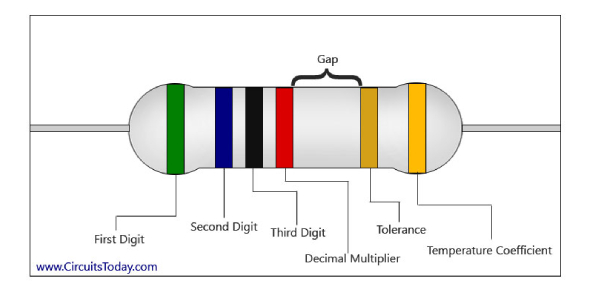# Quiz: Can You Decode Resistor Color Codes?

10 Questions | Attempts: 12717
ShareSettingsHow much do you know about the resistor color codes? Can you decode resistor color codes? This quiz will test your ability to decode a resistor's color code and calculate the resistance and tolerance of a resistor. This skill is needed by technicians, engineers, and some hobbyists. If you are one of them, the quiz will be helpful to you. Let's see if you can answer all the given questions correctly! We challenge you to get a good score; can you do it? Take our quiz & have fun!

• 1.
The resistor has 4 bands. Red, Green, Blue, Gold Which answer is correct?
• A.

25K Ohms with a 5% Tolerance and a 2.5K Ohm tolerance range

• B.

25M Ohms with a 5% Tolerance and a 2.5M Ohm tolerance range

• C.

6.5k Ohms with a 5% Tolerance and a 650 Ohm tolerance range

• D.

2500 Ohms with a 10% Tolerance and a 500 Ohm tolerance range

• E.

25M Ohms with a 10% Tolerance and a 5M Ohm tolerance range

• 2.
The resistor has 4 bands. Green, Blue, Red, Silver Which answer is correct?
• A.

56K Ohms with a 5% Tolerance and a 2.5K Ohm tolerance range

• B.

5.6K Ohms with a 5% Tolerance and a 560 Ohm tolerance range

• C.

56K Ohms with a 10 Tolerance and a 11.2K Ohm tolerance range

• D.

5.6K Ohms with a 10% Tolerance and a 1120 Ohm tolerance range

• E.

560 Ohms with a 5% Tolerance and a 56 Ohm tolerance range

• 3.
The resistor has 4 bands. Yellow, Brown, Brown, Gold Which answer is correct?
• A.

110k Ohms with a 5% Tolerance and a 11k Ohm tolerance range

• B.

410 Ohms with a 10% Tolerance and a 82 Ohm tolerance range

• C.

110k Ohms with a 10% Tolerance and a 22k Ohm tolerance range

• D.

4.1k Ohms with a 10% Tolerance and a 820 Ohm tolerance range

• E.

410 Ohms with a 5% Tolerance and a 41 Ohm tolerance range

• 4.
The resistor has 4 bands. Orange, Orange, Orange Which answer is correct?
• A.

33k Ohms with a 20% Tolerance and a 13.2k Ohm tolerance range

• B.

33k Ohms with a 10% Tolerance and a 6600 Ohm tolerance range

• C.

33k Ohms with a 5% Tolerance and a 3300 Ohm tolerance range

• D.

33k Ohms with a 2% Tolerance and a 660 Ohm tolerance range

• E.

33k Ohms with a 1% Tolerance and a 330 Ohm tolerance range

• 5.
The resistor has 4 bands. Brown, Back, Red, Gold Which answer is correct?
• A.

100 Ohms with a 1% Tolerance and a 2 Ohm tolerance range

• B.

1000 Ohms with a 5% Tolerance and a 100 Ohm tolerance range

• C.

10k Ohms with a 5% Tolerance and a 1K Ohm tolerance range

• D.

1000 Ohms with a 10% Tolerance and a 200 Ohm tolerance range

• E.

100 Ohms with a 20% Tolerance and a 40 Ohm tolerance range

• 6.
What is the resistivity of copper?
• A.

8 ohms

• B.

2. 4 ohms

• C.

10. 7 ohms

• D.

None of the above

• 7.
How is a fully functional 3.9 k ohms resistor color coded?
• A.

Red, green, orange, silver

• B.

Orange, green, orange, silver

• C.

Red, white, red, gold

• D.

Orange, white, red, gold

• 8.
The electrical component that is designed to resist the flow of the current?
• A.

Insulators

• B.

Resistors

• C.

Conductors

• D.

None of the above

• 9.
One can identify the wattage of a resistor by what?
• A.

Types of materials used

• B.

By it's internal construction

• C.

By it's size

• D.

Color code

• 10.
If the resistance of the system decreases, what happens to the current?
• A.

It stays the same

• B.

It decreases

• C.

It increases

• D.

None of the above

## Related TopicsBack to top
×

Wait!
Here's an interesting quiz for you.